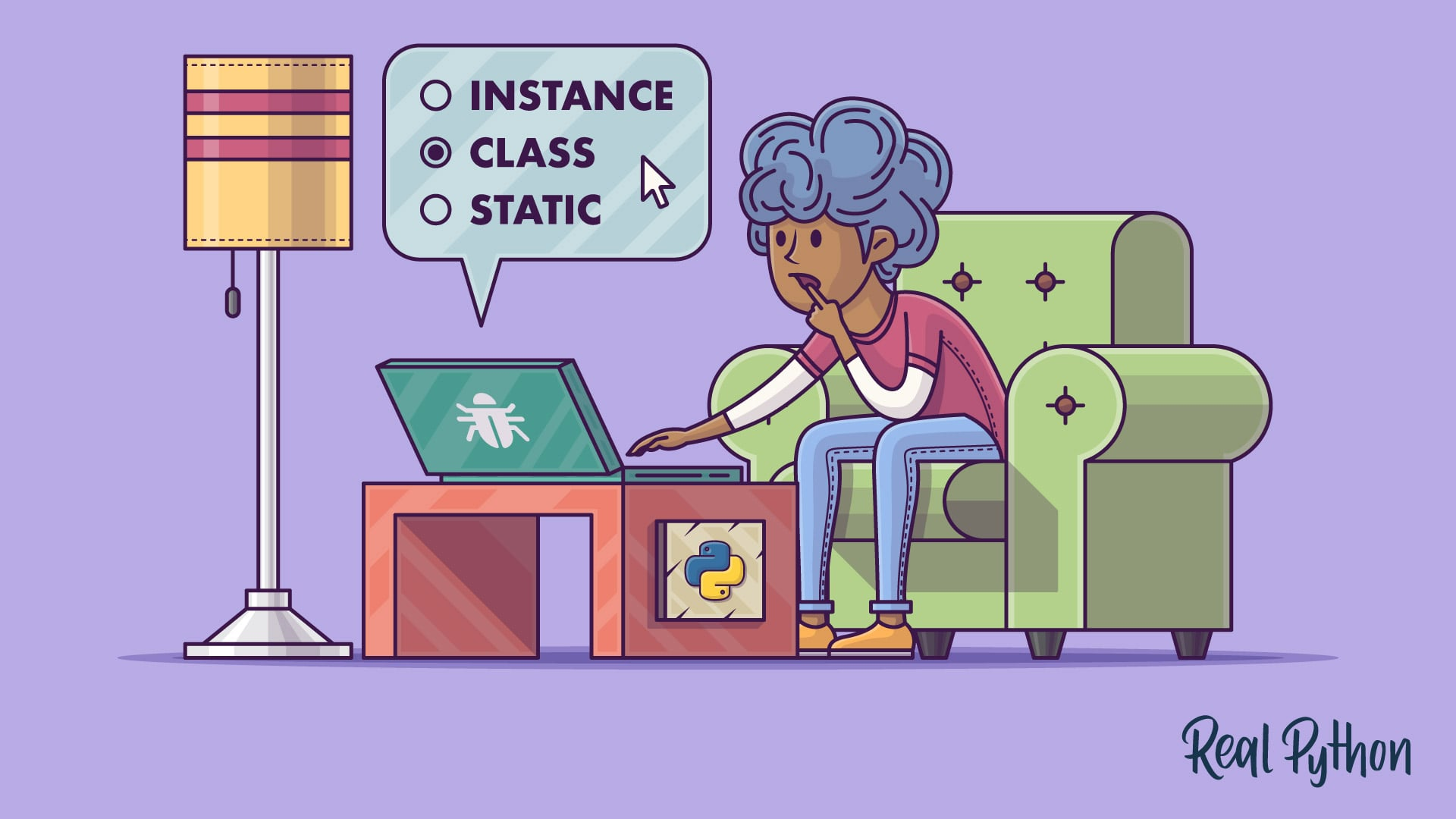物件的動作物件，動起來吧！

class dog {
constructor(n, a){      this.name = n;      this.age = a;   }}

dog1 = dog('Amy', 6);
dog2 = dog('Hank', 4);

print(dog1.name);
print(dog1.age);

class dog {
constructor(n, a){
this.name = n;
this.age = a;
}
selfIntroduce(){
print("I am " + this.name);
}
}

/* 建立物件 */
dog3 = dog('Sherry', 7);
/* 呼叫方法 */
dog3.selfIntroduce();
/* 螢幕上印出： */
/* 「I am Sherry」 */

用方法改變屬性

class dog{
constructor(n, a){
this.name = n;
this.age = a;
}
selfIntroduce(){
print("I am " + this.name);
}
birthday(){
this.age += 1;
}
}

dog4 = dog('Bob', 7);
print(dog4.age);
/* 螢幕上印出： */
/* 「7」 */
dog4.birthday();
print(dog4.age);
/* 螢幕上印出： */
/* 「8」 */

+1